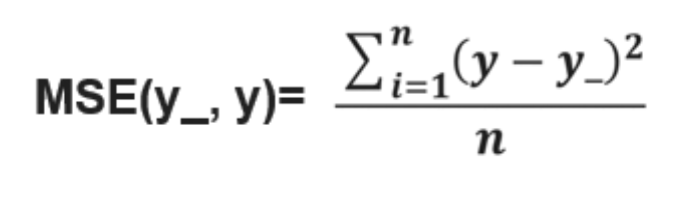# Tensorflow框架

## 人工智能实践：Tensorflow笔记（5）

Posted by     Keyon                      on March 28, 2018

## 张量、计算图、会话

``````with tf.Session() as sess:
print sess.run()
``````

## 神经网络

tf.random_normal() 生成正态分布随机数
tf.truncated_normal() 生成去掉过大偏离点的正态分布随机数
tf.random_uniform() 生成均匀分布随机数
tf.zeros 表示生成全 0 数组
tf.ones 表示生成全 1 数组
tf.fill 表示生成全定值数组
tf.constant 表示生成直接给定值的数组

1. 准备数据集，提取特征，作为输入喂给神经网络(Neural Network，NN)。
2. 搭建 NN 结构，从输入到输出(先搭建计算图，再用会话执行)( NN 前向传播算法 ➡️ 计算输出) 。
3. 大量特征数据喂给 NN，迭代优化 NN 参数( NN 反向传播算法 ➡️ 优化参数训练模型)。
4. 使用训练好的模型预测和分类。

• X：矩阵行数为输入特征的组数，列数为这组特征包含的元素种数。
• W：矩阵行数为前面节点数，列数为后面节点数。

``````with tf.Session() as sess:
sess.run()
``````

``````init_op = tf.global_variables_initializer()
sess.run(init_op)
````````````loss_mse = tf.reduce_mean(tf.square(y_ - y))
``````

``````train_step=tf.train.GradientDescentOptimizer(learning_rate).minimize(loss)
train_step=tf.train.MomentumOptimizer(learning_rate, momentum).minimize(loss)
``````

## 总结

``````	import
常量定义
生成数据集
``````

``````	x=  	y_=
w1=  	w2=
a=  	y=
``````

``````	loss=
train_step=
``````

``````with tf.session() as sess
Init_op=tf. global_variables_initializer()
sess_run(init_op)
STEPS=
for i in range(STEPS):
start=
end=
sess.run(train_step, feed_dict:)
``````